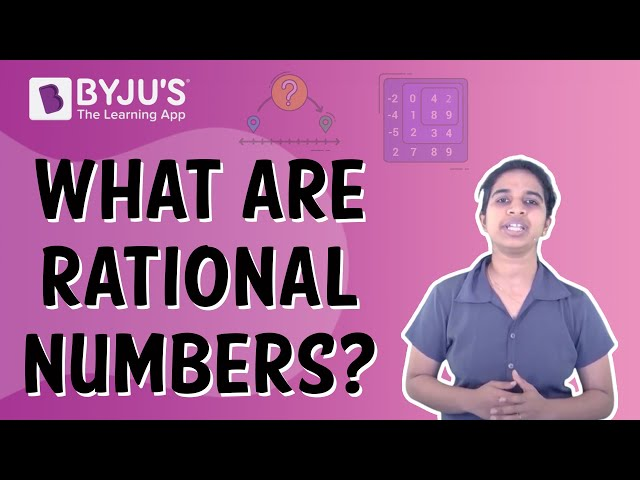# Rational Numbers Class 8

## Rational Numbers Class 8 Important concepts

In the number system, rational numbers are numbers that can be expressed as a ratio of two integers. They can also be the quotient of the ratio if the rational number is an integer. If the rational number is represented by the ratio p/q, then q must be a non-zero integer. Since the denominator can be 1, every integer is a rational number. In rational numbers class 8 covers all the concepts and the arithmetic operations and properties involved on rational numbers are clearly explained.

## Expression of integers as rational numbers

Any integer n can be expressed as a rational number n/1. This form is known as the canonical form of the integer.

Irreducible Fractions

All rational numbers can be expressed as an irreducible fraction x/y, where x and y are integers and y is not equal to zero. The canonical form of any rational number is expressed by dividing both the numerator and denominator by their greatest common divisor.

Equality of Two Rational Numbers

Two rational numbers x/y and a/b are said to be equal if:

x=a and y=b, as well as xb=ay.

Order of a Rational Number

A rational number a/b is said to be greater than x/y if and only if ay>bx.

Addition and Subtraction of Rational Numbers

Two rational numbers a/b and c/d are added as follows:

$$\begin{array}{l}\frac{a}{b}+\frac{c}{d} = \frac{ad+bc}{bd}\end{array}$$

Similarly the subtraction is done as:

$$\begin{array}{l}\frac{ad-bc}{bd}\end{array}$$

Multiplication of Rational Numbers

Two rational numbers a/b and c/d can be multiplied as ac/bd.

The rational numbers are in their canonical form, their product will be a reducible fraction.

Division of Rational Numbers

Division of rational numbers is carried out by multiplying one of the rational numbers with the reciprocal of the other.

To divide a/b and c/d, a/b is multiplied by d/c.

Inverse Numbers

All rational numbers have two inverses – additive inverse and multiplicative inverse.

Additive inverse of a/b  is – a/b and the multiplicative inverse is b/a.

## Video Lesson on Rational Numbers## Rational Numbers Class 8 Questions

Here are some practice questions in rational numbers class 8 will test your understanding of the concepts. All questions are objective type and you need to select the right option.

1. A number a/b is said to rational number if

1. Both a and b are integers
2. Both a and b are integers and b is not equal to zero
3. Both a and b are integers and a is not equal to zero
4. 4 Both a and b are integers and both a and b are not equal to zero

2. Which of the following statements is false?

1. Rational numbers are closed under addition
2. Rational numbers are closed under subtraction
3. Rational numbers are closed under multiplication
4. Rational numbers are closed under division

3. The multiplicative inverse of a negative rational number is

1. A negative rational number
2. A positive rational number
3. Zero
4. One

4. If a+0 = 0+a = a, which is a rational number, then 0 is called as:

1. Additional identity of rational numbers
2. Reciprocal of a
3. The multiplicative inverse of a